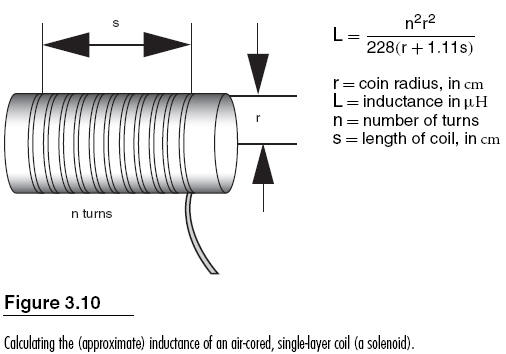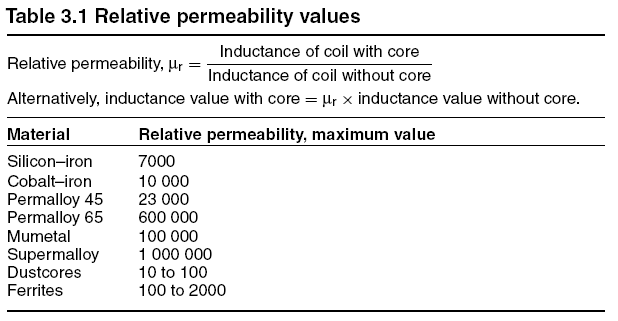Home | Gadget | Fuzzy | Lecturer  | Power  | Transceiver Find more type here... Inductance Calculations   Of all electronics calculations those of inductance are the least precise. When an air-cored coil is used, the changing magnetic field does not affect all of the turns equally, so only the central turns are fully affected with the outer turns receiving a lesser amount. Using a magnetic core concentrates the field so as to even out the effect, but also makes the size of induced EMF less predictable unless the effect of the core in terms of its relative permeability can be precisely measured. In addition, the relative permeability of the core changes if DC flows in the windings. We have seen also that effects such as skin effect and proximity effect will also require other corrections to calculations. Any equations for the inductance of a coil aretherefore very approximate and should be used only as a starting point in the construction of an inductor. Figure 3.10 shows a formula for the number of turns of a single-layer, close-wound coil, a solenoid to achieve a given inductance. The length of the coil is assumed to be more than the radius (otherwise a much more complex formula is needed). This approximate formula gives reasonable results for single-layer air-cored solenoids of the values that are used for tuning radio circuits at frequencies up to VHF, though a skin-effect correction may be needed at the higher frequencies. The addition of a core of ferrite material will cause an increase in inductance which could be by a factor as high as the relative permeability (Table 3.1) of the ferrite. Try http://www.vwlowen.demon.co.uk/java/coil.htm for finding number of turns of specified wire gauge to provide a stated inductance value, given diameter and length. The total permeability of a material is given by m0 × mr, where m0 is a universal constant called the permeability of free space, units henries per metre, and mr is relative permeability, a pure number with no units. These quantities are analogous to the permittivity of free space and relative permittivity as used for capacitor calculations.